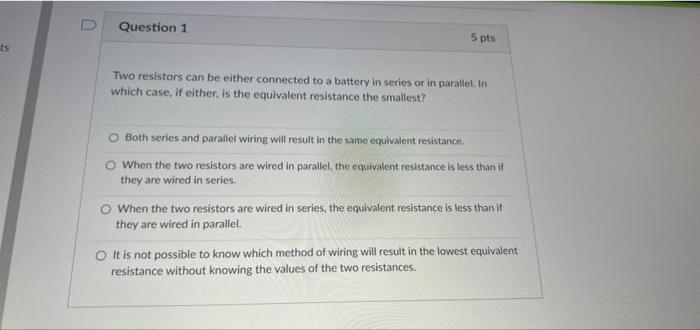# Question Question 1 5 pts ts Two resistors can be either connected to a battery in series or in parallel. In which case, if either, is the equivalent resistance the smallest? Both series and parallel wiring will result in the same equivalent resistance. When the two resistors are wired in parallel the equivalent resistance is less than it they are wired in series. o When the two resistors are wired in series, the equivalent resistance is less than it they are wired in parallel It is not possible to know which method of wiring will result in the lowest equivalent resistance without knowing the values of the two resistances.